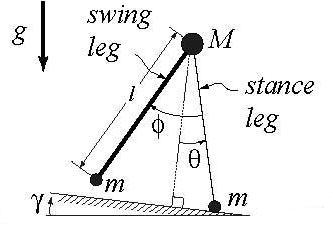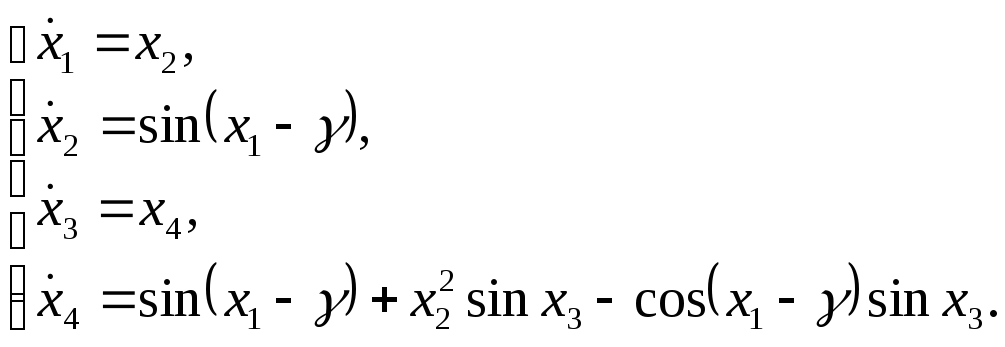# Б. О. Джолдошев а из Института автоматики и информационных технологий нан кр, г. Бишкек; «Cинтез кибернетических автоматических систем с использованием эталонной модели»

Главная страница
Контакты

Главная страница

##### Б. О. Джолдошев а из Института автоматики и информационных технологий нан кр, г. Бишкек; «Cинтез кибернетических автоматических систем с использованием эталонной модели»
 Скачать 28.25 Mb.

 страница 13/146 Дата 08.01.2017 Размер 28.25 Mb. Скачать 28.25 Mb.

Навигация по данной странице:
• Figure 9.

## Example

Double pendulum’s stable oscillations. As another example let us consider the dynamics of inverted double pendulum oscillations. For more simplicity let us take a model of simplest walker from  but assuming that one of the legs, namely stance leg, is fixed by its heel. The model is shown in the Fig. 9.

It has two rigid legs, connected by a frictionless hinge at a hip. Here is the angle of stance leg with respect to the slope normal, is the angle between the stance leg and the swing leg, is the ramp slope (which is used here only for the orientation of the pendulum in the space), g is the acceleration due to gravity.Figure 9. Double pendulum with slope

We assume that hip mass M is much larger than the foot mass m so that the motion of a swinging foot does not affect the motion of the hip. Finally, assuming that leg length l is equal to 9.8 m the dynamics of this construction is described by following equations(30)

where,, , andare denoted as x1, x2, x3, and x4 correspondingly.

If our task is to obtain a stable position of the stance leg and stable periodic oscillation of the swinging leg at possible uncertain perturbations of initial conditions and parameter of the system (30) in some ranges we can use the offered approach of using class of structurally stable functions from catastrophe theory.

Let us choose the ‘cusp’ catastrophe as a controller and take it without the germ• Figure 9.